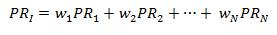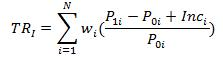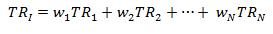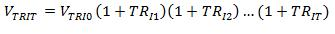### Why should I choose AnalystNotes?

AnalystNotes specializes in helping candidates pass. Period.

##### Subject 1. Index Definition and Calculations of Value and Returns

A security market index is a means to measure the value of a set of securities in a target market, market segment, or asset class. The constituent securities selected for inclusion in the security market index are intended to represent the target market.

There are usually two versions of the same index:

• The price return takes into account only the capital gain on an investment. A price return index reflects only the prices of the constituent securities. The income generated by the assets in the portfolio, in the form of interest and dividends, is ignored.

The value of a price return index is calculated as:ni: the number of units of security i in the index portfolio.
Pi: the unit price of security i.
D: the value of the divisor.

• The total return takes into account not only the capital appreciation on the portfolio, but also the income received. A total return index reflects the prices and the reinvestment of all income received since the inception of the index.

Single Period Returns

The single-period price return of an index is the weighted average of the price returns of the individual securities:orSince the total return of an index includes price appreciation and income, we need to add the weighted average of income to the above formula to calculate the single-period total return:orMultiple-Period Returns

The single-period returns should be linked geometrically.Similarly, to calculate the total return over multiple periods: